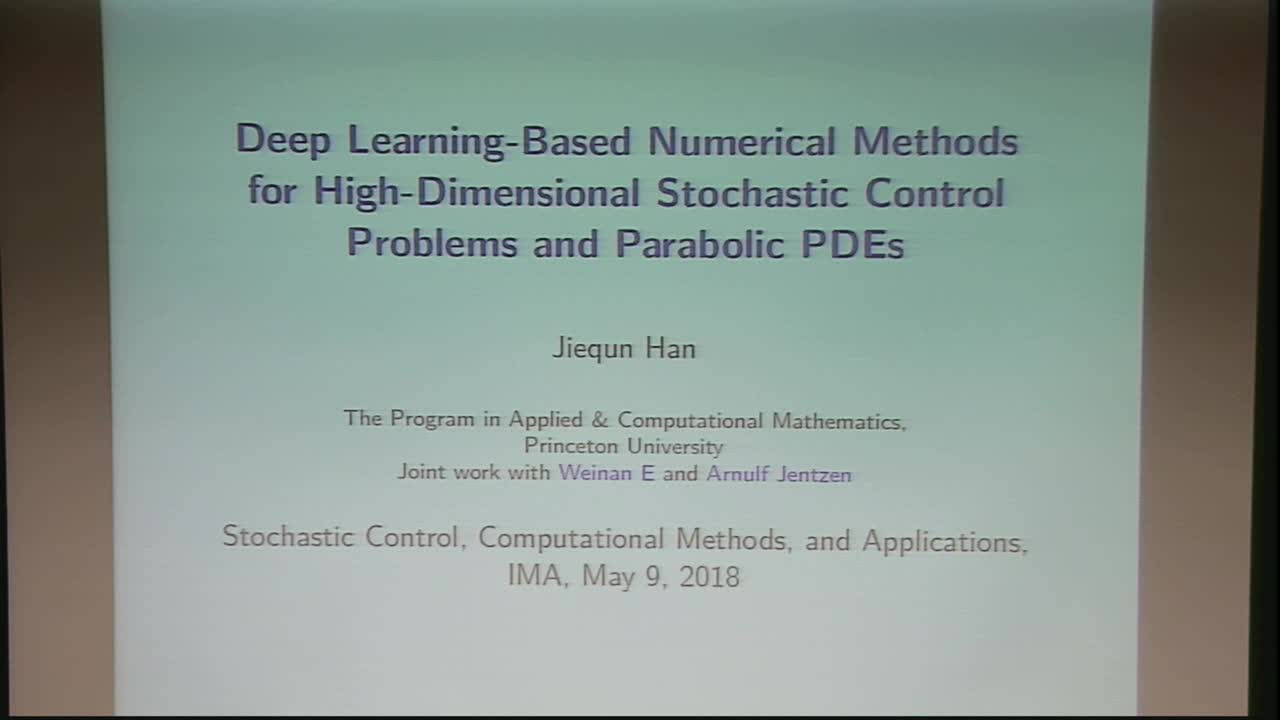## Deep Learning-Based Numerical Methods for High-Dimensional Stochastic Control Problems and Parabolic PDEs

May 9, 2018
IMA
Presenters: Jiequn Han

Watch Video

#### Abstract

Developing algorithms for solving high-dimensional stochastic control problems and high-dimensional partial differential equations (PDEs) has been an exceedingly difficult task for a long time, due to the notorious difficulty known as the "curse of dimensionality". In the first part of this talk, we develop a deep learning-based approach that directly solves high-dimensional stochastic control problems based on Monte-Carlo sampling. To this end, we approximate the time-dependent controls as feedforward neural networks and stack these networks together through model dynamics. In the second part of this talk, we propose the "deep BSDE method", in a similar vein, to solve general high-dimensional parabolic PDEs. In this context, the PDE is reformulated as a stochastic control problem through backward stochastic differential equation (BSDE) and the gradient of the unknown solution is approximated by neural networks. Numerical results of a variety of examples demonstrate that both algorithms are quite effective in high-dimensions, in terms of both accuracy and speed.